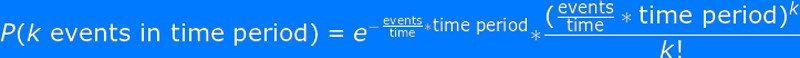# Which Type Of Queuing Model Would Be Best For Analyzing An Automated Car Wash?

Congestion at an automated car wash during peak hours can lead to customer frustration and loss of revenue. To lessen these problems, car wash business owners perform queuing analyses. These relied on certain analysis models.

Now, we would like to ask: What is the best type of queuing model to adapt for automated car wash businesses? We propose to use the Poisson model of distribution under the overarching umbrella of the theory called Queuing Theory. Here, you treat car arrivals as a Poisson process. That puts the variable “time between arrivals” as a distributed variable.

I remember that during my childhood, my parents often took me to the attic to observe meteor showers. Those precious bonding moments gazing at the night sky were my first encounter with the Poisson Analysis of Distribution. One cannot tell for sure how many cars will enter an automated car wash tunnel. In the same way, we also couldn’t predict when the next meteor would appear.

Now, I am a grown up with considerable data science skills. I now see that those “meteor events” and the times they appeared involved some mathematical laws or theories.

In this post, we will explore the topic more, and cover the following:

## How to Use Poisson for Car Wash Queuing Analysis?

The Poisson predicts the number of a particular type of event that will occur in a given period of time. For this theory to apply, the events should happen independently and not simultaneously. Using Poisson aims to find the probability of waiting some time until the next event. This is an analytical method appropriate for analyzing queuing in car wash businesses.

Here’s our working formula:Let’s say that the expected number (known average) of cars per hour is 5. Here, the given period is 1 and the expected number of cars to get a car wash service is 5.

The next step is to set another number (n) representing the number of cars that will actually get the service in one hour. For example, we ask: What is the probability that 2 cars will actually get the service in one hour? Here, k = 2.

This is our working equation:For simplicity, let’s compute first the value for (events/time) * time:

That is, 5/1 * 1 = 5.

Then:

P (2 cars will get the service in one hour) = e– (5) * [(5)2/2!) =0.00674 * (25/2) = 0.084224 or 8.42%.

Since the Poisson method is only applicable for discrete values, we use the value of 8, the nearest interest to the final result of our computation, and say that the probability of 2 cars getting a wash in a given period of 1 hour is 8%.

This means that in 100 observations, there will only be 8 times that there will be exactly 2 cars that will enter the wash tunnel and get a wash.

## Why Poisson for Car Wash Queuing Analysis?

We say that Poisson is the best applicable model for car wash queuing analysis because the queuing pattern in such businesses meets the following criteria:

• The events (someone getting a wash) can be counted in whole numbers.
• The frequency of occurrence in a given period is known.
• The events are independent.

To put it simply, if we are counting independent but identical events, then the events are likely to be a Poisson process.

## Limitations of Poisson

One disadvantage of the Poisson is that it makes a strong assumption as regards the distribution of the underlying data. In particular, the theory assumes that the mean (average) is equal to the variance. The assumption is applicable in some situations, but may not be appropriate for other situations.

Another point of weakness for Poisson is that little is known of the upper limit where the theory fails to be a good approximation. In car wash businesses, the Poisson approximation is only valid for conditions up to the full capacity of the facility. Here, the value of the variance becomes much greater than the mean. When this happens, one can resort to using the normal distribution analysis, which is more appropriately applicable.

## Final Thoughts

We are looking for the best theoretical model to use in car wash queuing analyses to prevent revenue loss and improve customer satisfaction. Car arrivals are independent of each other and these events are identical. These conditions are necessary for using Poisson. Thus, we recommend the use of this analytical method. The Poisson analytical approach is one of the easiest methods of analysis for data distribution.

The next step is improving your queuing management. Now that you are able to predict (using Poisson) when your facility will have a number of customers at a particular time, you can get your staff and facility ready for them and for that particular time.

When the line becomes longer than you expected, you can always use certain strategies. These include keeping the waiting customers informed and busy. Have little conversations with them, and take advantage of the time to promote your business.

Show them that you care. As soon as the tunnel becomes vacated, move fast, and start the wash right away.

Categories FAQ